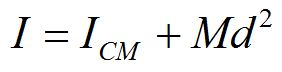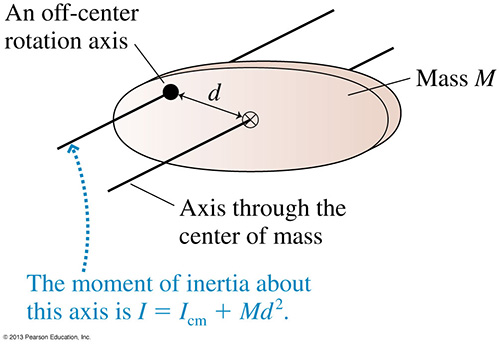PH 212

Rigid rotational motion

• Center of mass
• Rotational energy
• Moment of inertia
• Torque
• Rotational dynamics
• Fixed axis
• Static equilibrium
• Rolling
• Vector description
• Angular momentum conservation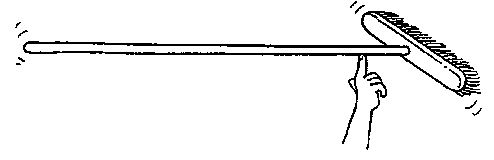© 2005 Pearson Prentice Hall, Inc

The broom balances at its center of mass. If you saw the broom through its center of mass and weigh the two parts, which part will weigh more?

An unconstrained object rotates about its center of mass.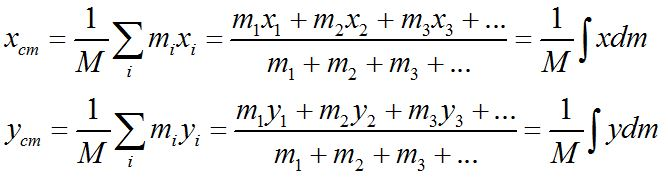A rotating object has kinetic energy due to the motion of its particles.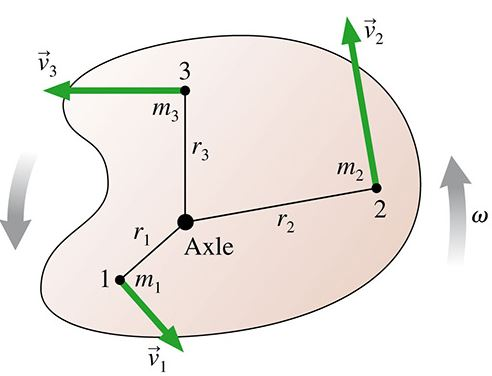© 2013 Pearson Education, Inc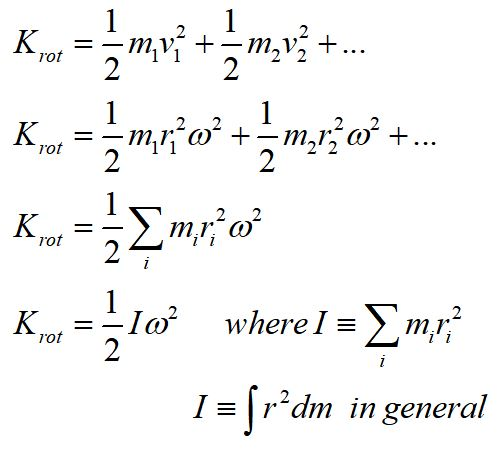Note that the radius for each mass element is the distance of the mass element from the axis of rotation.

The moment of inertia, I, is a measure of the mass distribution of a rotating object. Its definition depends on the axis of rotation. A larger moment of inertia means an object needs more torque to produce an angular acceleration.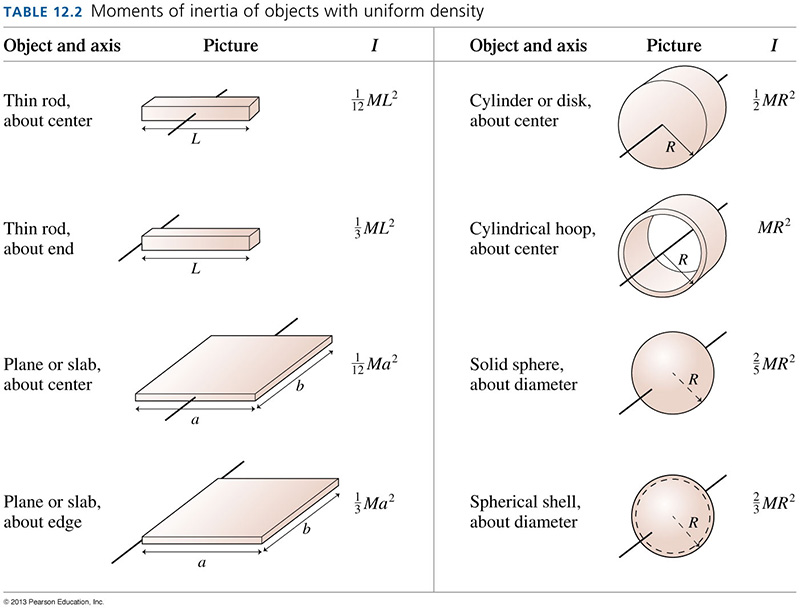Using the concept of the moment of inertia, which do you think would be easier to balance, a hammer with head up or a hammer with head down?

A. head up

B. head down

C. they would be the same

Parallel axis theorem

If an object is made to rotate about an axis that does not go through the center of mass, the moment of inertia can be found using the parallel axis theorem.